Definitions of Square Dance Calls and Concepts
Lock 'Em Up [C3B]Index -->  Plus  |  A1  |  A2  |  C1  |  C2  |  C3A  |  C3B  |  C4  |  NOL  |
Definitions (Text Only) -->  Plus  |  A1  |  A2  |  C1  |  C2  |  C3A  |  C3B  |  C4  |  NOL  |
 Find call:

 \$B8@8l(B\$BE,@Z\$J(B Tidal Line \$B\$^\$?\$O(B, \$BE,@Z\$JJ?9T\$J(B Line \$B\$+\$i(B.

1. Lockit;
2. Hinge;
3. Centers \$B\$O(B Hinge \$B\$r(B, Outsides \$B\$O(B Counter Rotate 1/4 \$B\$r(B;
4. Centers \$B\$O(B Lockit \$B\$r(B, Outsides \$B\$O(B Roll \$B\$r\$7\$^\$9(B.

4 \$B%Q!<%H\$N%3!<%k\$G\$9(B.

Lock 'Em Up \$B\$N3P\$(\$d\$9\$\$J}K!(B:
Lock The Hinge \$B\$r\$7\$F(B; Centers \$B\$O(B Hinge the Lock \$B\$r(B, Ends \$B\$O(B Counter Rotate 1/4 & Roll \$B\$r\$7\$^\$9(B.

From a Tidal Wave: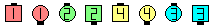Lock 'Em Up\$B\$NA0(B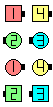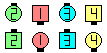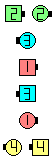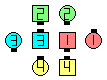Lockit(1/4)\$B\$N8e(B Hinge(1/2)\$B\$N8e(B Centers Hinge as Outsides Counter Rotate 1/4(3/4)\$B\$N8e(B Centers Lockit as Outsides Roll\$B\$N8e(B (\$B=*\$o\$j(B)

From Parallel Two-Faced Lines: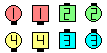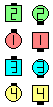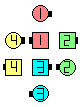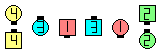Lock 'Em Up\$B\$NA0(B Lock The Hinge(1/2)\$B\$N8e(B Centers Hinge as Outsides Counter Rotate 1/4(3/4)\$B\$N8e(B Centers Lockit as Outsides Roll\$B\$N8e(B (\$B=*\$o\$j(B)

Cross Lock 'Em Up [C3BV]: Cross Lockit; Hinge; Centers \$B\$O(B Hinge \$B\$r(B, Outsides \$B\$O(B Counter Rotate 1/4 \$B\$r\$7(B; Centers \$B\$O(B Lockit \$B\$r(B, Outsides \$B\$O(B Roll \$B\$r\$7\$^\$9(B.

anything 'Em Up [C3BV]: Hinge; Centers \$B\$O(B Hinge \$B\$r(B, Outsides \$B\$O(B Counter Rotate 1/4 \$B\$r\$7(B; Centers \$B\$O(B Lockit \$B\$r(B, Outsides \$B\$O(B Roll \$B\$r\$7\$^\$9(B. \$B\$9\$J\$o\$A(B, Lock 'Em Up \$B\$N8e\$N(B 3/4 \$B\$r\$7\$^\$9(B.Choreography for Lock 'Em UpComments? Questions? Suggestions?